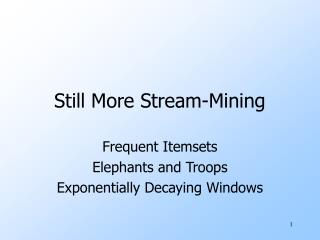# Still More Stream-Mining - PowerPoint PPT PresentationDownload PresentationStill More Stream-Mining

Still More Stream-Mining
Download Presentation## Still More Stream-Mining

- - - - - - - - - - - - - - - - - - - - - - - - - - - E N D - - - - - - - - - - - - - - - - - - - - - - - - - - -
##### Presentation Transcript

1. Still More Stream-Mining Frequent Itemsets Elephants and Troops Exponentially Decaying Windows

2. Counting Items • Problem: given a stream, which items appear more than s times in the window? • Possible solution: think of the stream of baskets as one binary stream per item. • 1 = item present; 0 = not present. • Use DGIM to estimate counts of 1’s for all items.

3. Extensions • In principle, you could count frequent pairs or even larger sets the same way. • One stream per itemset. • Drawbacks: • Only approximate. • Number of itemsets is way too big.

4. Approaches • “Elephants and troops”: a heuristic way to converge on unusually strongly connected itemsets. • Exponentially decaying windows: a heuristic for selecting likely frequent itemsets.

5. Elephants and Troops • When Sergey Brin wasn’t worrying about Google, he tried the following experiment. • Goal: find unusually correlated sets of words. • “High Correlation ” = frequency of occurrence of set >> product of frequency of members.

6. Experimental Setup • The data was an early Google crawl of the Stanford Web. • Each night, the data would be streamed to a process that counted a preselected collection of itemsets. • If {a, b, c} is selected, count {a, b, c}, {a}, {b}, and {c}. • “Correlation” = n2 * #abc/(#a * #b * #c). • n = number of pages.

7. After Each Night’s Processing . . . • Find the most correlated sets counted. • Construct a new collection of itemsets to count the next night. • All the most correlated sets (“winners ”). • Pairs of a word in some winner and a random word. • Winners combined in various ways. • Some random pairs.

8. After a Week . . . • The pair {“elephants”, “troops”} came up as the big winner. • Why? It turns out that Stanford students were playing a Punic-War simulation game internationally, where moves were sent by Web pages.

9. Mining Streams Vs. Mining DB’s (New Topic) • Unlike mining databases, mining streams doesn’t have a fixed answer. • We’re really mining in the “Stat” point of view, e.g., “Which itemsets are frequent in the underlying model that generates the stream?”

10. Stationarity • Two different assumptions make a big difference. • Is the model stationary ? • I.e., are the same statistics used throughout all time to generate the stream? • Or does the frequency of generating given items or itemsets change over time?

11. Some Options for Frequent Itemsets • We could: • Run periodic experiments, like E&T. • Like SON --- itemset is a candidate if it is found frequent on any “day.” • Good for stationary statistics. • Frame the problem as finding all frequent itemsets in an “exponentially decaying window.” • Good for nonstationary statistics.

12. Exponentially Decaying Windows • If stream is a1, a2,… and we are taking the sum of the stream, take the answer at time t to be:Σi = 1,2,…,t ai e -c (t-i). • c is a constant, presumably tiny, like 10-6 or 10-9.

13. Example: Counting Items • If each aiis an “item” we can compute the characteristic function of each possible item x as an E.D.W. • That is: Σi = 1,2,…,tδi e -c (t-i), whereδi = 1 if ai= x, and 0 otherwise. • Call this sum the weight of item x.

14. Counting Items --- (2) • Suppose we want to find those items of weight at least ½. • Important property: sum over all weights is e c/(e c – 1) or very close to 1/c. • Thus: at most 2/c items have weight at least ½.

15. Extension to Larger Itemsets* • Count (some) itemsets in an E.D.W. • When a basket B comes in: • Multiply all counts by (1-c ); drop counts < ½. • If an item in B is uncounted, create new count. • Add 1 to count of any item in B and to any counted itemset contained in B. • Initiate new counts (next slide). * Informal proposal of Art Owen

16. Initiation of New Counts • Start a count for an itemset S ⊆B if every proper subset of S had a count prior to arrival of basket B. • Example: Start counting {i, j } iff both i and j were counted prior to seeing B. • Example: Start counting {i, j, k } iff {i, j }, {i, k }, and {j, k } were all counted prior to seeing B.

17. How Many Counts? • Counts for single items = (2/c ) times the average number of items in a basket. • Counts for larger itemsets = ??. But we are conservative about starting counts of large sets. • If we counted every set we saw, one basket of 20 items would initiate 1M counts.